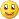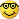# Multi Timeframe Indicators - page 553254

Marco The old sentence (heard so many times) : that is decompiled code. Sorry

Ok thx. I can't see if something is decompiled, how can I see this so I won't have to send this stuff anymore!

Thx and regards Marco153912

Marco320:

Ok thx. I can't see if something is decompiled, how can I see this so I won't have to send this stuff anymore!

Thx and regards MarcoMarco

Here is one post about that : https://www.mql5.com/en/forum/general

regards153912

Marco

Just an unrelated example : take a look at a part of JMA that is floating around for years and is used for years

ring1 [counterA] = highDValue;

if ((limitValue + startValue) > 127) {

startValue--;

s58 = startValue;

} else {

limitValue++;

s58 = limitValue;

}

if (limitValue > 96) s38 = 96; else s38 = limitValue;

if (startValue < 32) s40 = 32; else s40 = startValue;

}

//----

s68 = 64;

s60 = s68;

while (s68 > 1) {

if (list [s60] >= highDValue) {

if (list [s60 - 1] <= highDValue) {

s68 = 1;

}

else {

s68 = s68 / 2.0;

s60 -= s68;

}

}

else {

s68 = s68 / 2.0;

s60 += s68;

}

if ((s60 == 127) && (highDValue > list)) s60 = 128;

}

if (cycleLimit > 127) {

if (s58 >= s60) {

if (((s38 + 1) > s60) && ((s40 - 1) < s60))

lowDValue += highDValue;

else if ((s40 > s60) && ((s40 - 1) < s58))

lowDValue += list [s40 - 1];

}

else if (s40 >= s60) {

if (((s38 + 1) s58))

lowDValue += list[s38 + 1];

}

else if ((s38 + 2) > s60)

lowDValue += highDValue;

else if (((s38 + 1) s58))

lowDValue += list[s38 + 1];

if (s58 > s60) {

if (((s40 - 1) s58))

lowDValue -= list [s58];

else if ((s38 s60))

lowDValue -= list [s38];

}

else {

if (((s38 + 1) > s58) && ((s40 - 1) < s58))

lowDValue -= list [s58];

else if ((s40 > s58) && (s40 < s60))

lowDValue -= list [s40];

}

}

That is a decompiled Wealth lab code (decompiled years ago) that was converted to metatrader. I have seen some code written by Mark Jurik and he never ever used names like that for variables, but he uses normal, human names. So I guess that if the start was like that (since JMA became popular right at the beginning of metatrader) we could not expect anything different when it comes to metatrader itself

So that is not a game invented by metatrader users but it seems that they are the ones that are killing the coding by flooding the net with decompiled code which makes the coders not post anything any more. and since the majority simply are not coders they even do not know when the code is decompiled - and the rest is known254

Marco

Just an unrelated example : take a look at a part of JMA that is floating around for years and is used for years

ring1 [counterA] = highDValue;

if ((limitValue + startValue) > 127) {

startValue--;

s58 = startValue;

} else {

limitValue++;

s58 = limitValue;

}

if (limitValue > 96) s38 = 96; else s38 = limitValue;

if (startValue < 32) s40 = 32; else s40 = startValue;

}

//----

s68 = 64;

s60 = s68;

while (s68 > 1) {

if (list [s60] >= highDValue) {

if (list [s60 - 1] <= highDValue) {

s68 = 1;

}

else {

s68 = s68 / 2.0;

s60 -= s68;

}

}

else {

s68 = s68 / 2.0;

s60 += s68;

}

if ((s60 == 127) && (highDValue > list)) s60 = 128;

}

if (cycleLimit > 127) {

if (s58 >= s60) {

if (((s38 + 1) > s60) && ((s40 - 1) < s60))

lowDValue += highDValue;

else if ((s40 > s60) && ((s40 - 1) < s58))

lowDValue += list [s40 - 1];

}

else if (s40 >= s60) {

if (((s38 + 1) s58))

lowDValue += list[s38 + 1];

}

else if ((s38 + 2) > s60)

lowDValue += highDValue;

else if (((s38 + 1) s58))

lowDValue += list[s38 + 1];

if (s58 > s60) {

if (((s40 - 1) s58))

lowDValue -= list [s58];

else if ((s38 s60))

lowDValue -= list [s38];

}

else {

if (((s38 + 1) > s58) && ((s40 - 1) < s58))

lowDValue -= list [s58];

else if ((s40 > s58) && (s40 < s60))

lowDValue -= list [s40];

}

}

That is a decompiled Wealth lab code (decompiled years ago) that was converted to metatrader. I have seen some code written by Mark Jurik and he never ever used names like that for variables, but he uses normal, human names. So I guess that if the start was like that (since JMA became popular right at the beginning of metatrader) we could not expect anything different when it comes to metatrader itself

So that is not a game invented by metatrader users but it seems that they are the ones that are killing the coding by flooding the net with decompiled code which makes the coders not post anything any more. and since the majority simply are not coders they even do not know when the code is decompiled - and the rest is known

That's right, some people (the wild guru stories e.g.) takes the wrong step by selling stolen or existing products (even a lot is free to get,) and the web is that big so you can find a lot. It's not the product you sell, but the input of parameters which can make a system and then recognize how to trade with.

Indeed I'm not a coder, basics are simple to change, but the real work, I'm glad e.g. you and mrTools are here to help. I will take more care to look if I will recognize a normal code or decompiled, so it safe time for both of us. It's a sort of scholing for me, little by little learning more of MQL. So, thx for the briefing and till soon!!!

Best regards, Marco113

Kelch Monteiro It needs "ang_AZad[Osc]" indicator in the indicators folder in order to work. Without it you will get an empty sub-window

Mladen, is possible make it with the "ki" fractionated, like 1.5 or 3.6 periods?9492

Kelch Monteiro:
Mladen, is possible make it with the "ki" fractionated, like 1.5 or 3.6 periods?

Kelch Monteiro, in the code change, extern int ki = 2; to extern double ki = "whatever fractional number"; Change the int to double, that's all.101

Can anyone turn it into a non-lagging MTF version?( i mean non-adjusting mtf indicator showing real values of higher timeframes )

Running Median Indicator for MT4 - MQL4 Code Base67

Many thanks. Mladen and Marco...I will give it a try113

mrtools:
Kelch Monteiro, in the code change, extern int ki = 2; to extern double ki = "whatever fractional number"; Change the int to double, that's all.

thanks man! can you indicate some good and easy e-book to me? I really would like to learn mql4. i already searched on net but the e-books what I found needs to already know something about programming. I don't even know about html. lol some e-book to learn from begin. can you indicate?9492

Kelch Monteiro:
thanks man! can you indicate some good and easy e-book to me? I really would like to learn mql4. i already searched on net but the e-books what I found needs to already know something about programming. I don't even know about html. lol some e-book to learn from begin. can you indicate?

Kelch Monteiro, there is a tutorial zip file on this link https://www.mql5.com/en/forum/176544 if you haven't already done so would recommend you read it, and do the simple little exercises and practice the coding, it will eventually come, because believe me if I can do it, think anyone can.